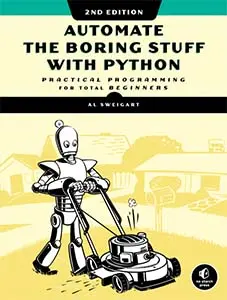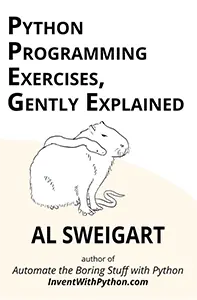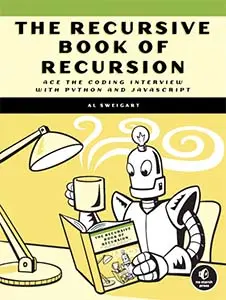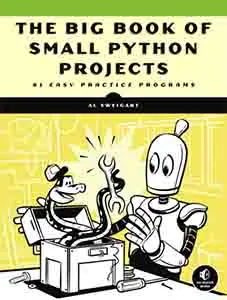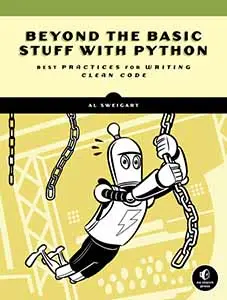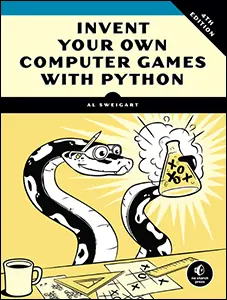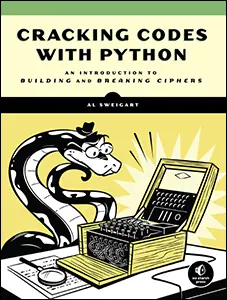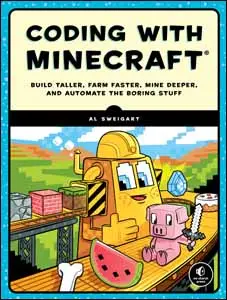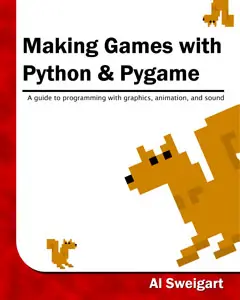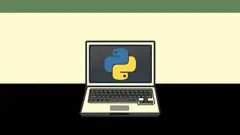# The Invent with Python Blog

Writings from the author of Automate the Boring Stuff.

Sun 05 September 2021

# Recursion Is Not A Superpower: An Iterative Ackermann

Posted by Al Sweigart in python

This post has the Python code for the infamously recursive Ackermann function, but without using recursion. Many people think that recursive algorithms hold some special power that can do computations beyond the reach of iterative algorithms. But recursion is not a superpower: there's nothing you can do with recurison that you can't do with a loop and a stack.

This blog post contains information from Al Sweigart's next book, The Recursive Book of Recurson from No Starch Press. The book will be released in February 2022. You can use the discount code PREORDERRECURSION to get 30% off your preorder, and receive the ebook free when you buy a print book. This book will also be freely available under a Creative Commons license on the Invent with Python website. Al Sweigart also gave a conference talk on recursion at North Bay Python 2018 titled, Recursion for Beginners: A Beginner's Guide to Recursion.

A stack is one of the simplest data structures in computing. It stores multiple values like a list or an array does, but unlike lists or arrays, it limits you to adding to or removing values from the “top” of the stack only. Adding values is called pushing values onto the stack, while removing values is called popping values off the stack.

The call stack is a stack data structure your programming language's compiler or interpreter uses to keep track of where in your program the execution should return to when a function call finishes. When your program calls a function, this return address information is pushed onto the stack. When the function call returns, the information is popped off the stack. If your function call makes a function call which makes another function call, you'll have three things pushed onto the call stack.

Recursion is confusing for beginner because instructors and tutorials often don't explicitly explain what the call stack is. The call stack is managed by the compiler or interpreter automatically in the background. You can't point to a place in your source code and say, "this is the call stack" like you can with other variables. This makes the call stack an invisible gap in the understanding of students.

Recursive functions use the call stack as their stack data structure, but you can use a list or array as a stack data structure by restricting yourself to adding or removing things only from the end. For example, Python's `append()` and `pop()` list methods are effectively the "push" and "pop" operations to treat a Python list as a stack.

There is nothing special about recursion. Anything you can do with recursion can be done with a loop and a stack. Further, recursion is not automatically more "elegant" than an iterative solution. Quite the opposite: recursion is often produces overengineered and less readable code than a straightforward iterative solution.

To prove this, I'll use the famous Ackermann recursive function as an example. The Ackermann function doesn't have much practical use aside from an example of a recursive function that is extremely recursive. Even small inputs to this function can result in an amount of computation that takes years or lifetimes to finish. Here's the code to the recursive Ackermann function in Python:

```def ackermann(m, n):
if m == 0:
# BASE CASE
return n + 1
elif m > 0 and n == 0:
# RECURSIVE CASE
return ackermann(m - 1, 1)
elif m > 0 and n > 0:
# RECURSIVE CASE
return ackermann(m - 1, ackermann(m, n - 1))```

For example, calling `ackermann(1, 1)` will take less than a second to complete. Calling `ackermann(4,1)` will take a couple minutes. But calling `ackermann(15, 20)` will take longer than the universe has existed to finish calculating. The Ackermann function becomes untennable very quickly.

But recursion is not a superpower. Even Ackermann, one the most recursive of recursive functions, can be written with a loop and a stack. Here's an iterative Ackermann implementation in Python:

```print('Starting with m = 2, n = 3:')
callStack = [{'m': 2, 'n': 3, 'indentation': 0, 'instrPtr': 'start'}]
returnValue = None

while len(callStack) != 0:
m = callStack[-1]['m']
n = callStack[-1]['n']
indentation = callStack[-1]['indentation']
instrPtr = callStack[-1]['instrPtr']

if instrPtr == 'start':
print('%sackermann(%s, %s)' % (' ' * indentation, m, n))

if m == 0:
# BASE CASE
returnValue = n + 1
callStack.pop()
continue
elif m > 0 and n == 0:
# RECURSIVE CASE
callStack[-1]['instrPtr'] = 'after first recursive case'
callStack.append({'m': m - 1, 'n': 1, 'indentation': indentation + 1, 'instrPtr': 'start'})
continue
elif m > 0 and n > 0:
# RECURSIVE CASE
callStack[-1]['instrPtr'] = 'after second recursive case, inner call'
callStack.append({'m': m, 'n': n - 1, 'indentation': indentation + 1, 'instrPtr': 'start'})
continue
elif instrPtr == 'after first recursive case':
returnValue = returnValue
callStack.pop()
continue
elif instrPtr == 'after second recursive case, inner call':
callStack[-1]['innerCallResult'] = returnValue
callStack[-1]['instrPtr'] = 'after second recursive case, outer call'
callStack.append({'m': m - 1, 'n': returnValue, 'indentation': indentation + 1, 'instrPtr': 'start'})
continue
elif instrPtr == 'after second recursive case, outer call':
returnValue = returnValue
callStack.pop()
continue
print(returnValue)```

Try running both programs. You'll see that they produce identical output. But the iterative version doesn't use recursive functions. In fact, it doesn't use functions at all: there's no `def` statement anywhere in the program.

If you find recursion confusing or wonder why you "should" use recursion, don't despair. Recursion is an inappropriate approach just as often as it is appropriate (or even more). Just remember that recursion, while a useful technique that every programmer should understand, is not a superpower.

If you'd like to learn how to use recursion, check out Al Sweigart's upcoming book, The Recursive Book of Recursion from No Starch Press. This book will also be freely available under a Creative Commons license on the Invent with Python website. Al Sweigart also gave a conference talk on recursion at North Bay Python 2018 titled, Recursion for Beginners: A Beginner's Guide to Recursion.